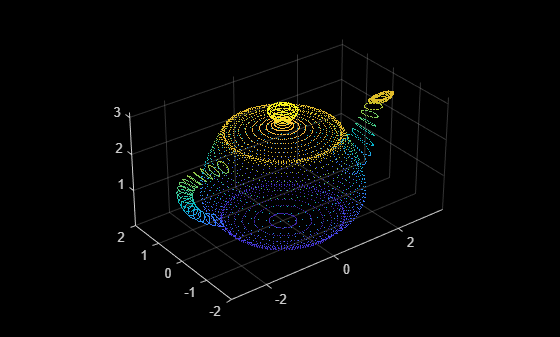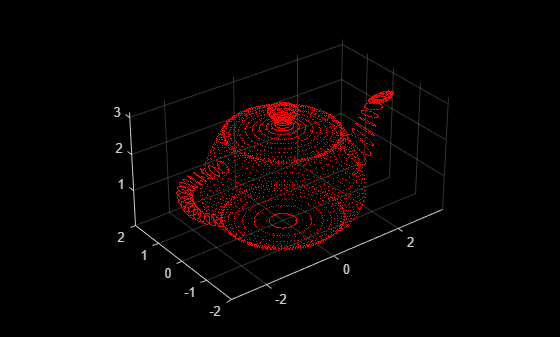# pointCloud

Object for storing 3-D point cloud

## Description

The `pointCloud` object creates point cloud data from a set of points in 3-D coordinate system. The point cloud data is stored as an object with the properties listed in Properties. Use Object Functions to retrieve, select, and remove desired points from the point cloud data.

## Creation

### Syntax

``ptCloud = pointCloud(xyzPoints)``
``ptCloud = pointCloud(xyzPoints,Name,Value)``

### Description

````ptCloud = pointCloud(xyzPoints)` returns a point cloud object with coordinates specified by `xyzPoints`.```

example

````ptCloud = pointCloud(xyzPoints,Name,Value)` creates a `pointCloud` object with properties specified as one or more `Name,Value` pair arguments. For example, `pointCloud(xyzPoints,'Color',[0 0 0])` sets the `Color` property of the point `xyzPoints` as [0 0 0]. Enclose each property name in quotes. Any unspecified properties have default values.```

### Input Arguments

expand all

3-D coordinate points, specified as an M-by-3 list of points or an M-by-N-by-3 array for an organized point cloud. The 3-D coordinate points specify the x, y, and z positions of a point in the 3-D coordinate space. The first two dimensions of an organized point cloud correspond to the scanning order from sensors such as RGBD or lidar. This argument sets the `Location` property.

Data Types: `single` | `double`

### Output Arguments

expand all

Point cloud, returned as a `pointCloud` object with the properties listed in Properties.

## Properties

expand all

Position of the points in 3-D coordinate space, specified as an M-by-3 or M-by-N-by-3 array. Each entry specifies the x, y, and z coordinates of a point in the 3-D coordinate space. You cannot set this property as a name-value pair. Use the `xyzPoints` input argument.

Data Types: `single`

Point cloud color, specified as an M-by-3 or M-by-N-by-3 array. Use this property to set the color of points in point cloud. Each entry specifies the RGB color of a point in the point cloud data. Therefore, you can specify the same color for all points or a different color for each point.

• The specified RGB values must lie within the range [0, 1], when you specify the data type for `Color` as `single` or `double`.

• The specified RGB values must lie within the range [0, 255], when you specify the data type for `Color` as `uint8`.

 Coordinates Valid assignment of `Color` M-by-3 array M-by-3 array containing RGB values for each pointM-by-N-by-3 array M-by-N-by-3 array containing RGB values for each pointData Types: `uint8`

Surface normals, specified as a M-by-3 or M-by-N-by-3 array. Use this property to specify the normal vector with respect to each point in the point cloud. Each entry in the surface normals specifies the x, y, and z component of a normal vector.

CoordinatesSurface Normals
M-by-3 arrayM-by-3 array, where each row contains a corresponding normal vector.
M-by-N-by-3 arrayM-by-N-by-3 array containing a 1-by-1-by-3 normal vector for each point.

Data Types: `single` | `double`

Grayscale intensities at each point, specified as a M-by-1 vector or M-by-N matrix. The function maps each intensity value to a color value in the current colormap.

CoordinatesIntensity
M-by-3 arrayM-by-1 vector, where each row contains a corresponding intensity value.
M-by-N-by-3 arrayM-by-N matrix containing intensity value for each point.

Data Types: `single` | `double` | `uint8`

Number of points in the point cloud, stored as a positive integer.

Range of coordinates along x-axis, stored as a 1-by-2 vector.

Range of coordinates along y-axis, stored as a 1-by-2 vector.

Range of coordinates along z-axis, stored as a 1-by-2 vector.

## Object Functions

 `findNearestNeighbors` Find nearest neighbors of a point in point cloud `findNeighborsInRadius` Find neighbors within a radius of a point in the point cloud `findPointsInROI` Find points within a region of interest in the point cloud `removeInvalidPoints` Remove invalid points from point cloud `select` Select points in point cloud `copy` Copy array of handle objects

## Examples

collapse all

Read the 3-D coordinate points into the workspace.

`load('xyzPoints');`

Create a point cloud object from the input point coordinates.

`ptCloud = pointCloud(xyzPoints);`

Inspect the properties of the point cloud object.

`ptCloud`
```ptCloud = pointCloud with properties: Location: [5184×3 single] Color: [] Normal: [] Intensity: [] Count: 5184 XLimits: [-3 3.4338] YLimits: [-2 2] ZLimits: [0.0016 3.1437] ```

Display the point cloud by using `pcshow`.

`pcshow(ptCloud)`Modify Color of Point Cloud Data

Create an RGB color array of size same as the size of the point cloud data. Set the point colors to Red.

`cmatrix = ones(size(ptCloud.Location)).*[1 0 0];`

Create the point cloud object with the color property set to the RGB color array.

```ptCloud = pointCloud(xyzPoints,'Color',cmatrix); pcshow(ptCloud)```Add Surface Normals to Point Cloud Data

Compute surface normals corresponding to the point cloud data using `pcnormals`.

`normals = pcnormals(ptCloud);`

Create point cloud object from input point coordinates. Add the computed surface normals to point cloud object.

`ptCloud = pointCloud(xyzPoints,'Normal',normals);`

Display the point cloud and plot the surface normals.

```pcshow(ptCloud) x = ptCloud.Location(:,1); y = ptCloud.Location(:,2); z = ptCloud.Location(:,3); u = normals(:,1); v = normals(:,2); w = normals(:,3); hold on quiver3(x,y,z,u,v,w); hold off```## Tips

The `pointCloud` object is a `handle` object. If you want to create a separate copy of a point cloud, you can use the MATLAB® `copy` method.

 `ptCloudB` = `copy`(`ptCloudA`)

If you want to preserve a single copy of a point cloud, which can be modified by point cloud functions, use the same point cloud variable name for the input and output.

 `ptCloud` = pcFunction(`ptCloud`)